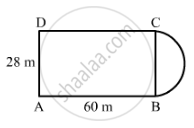Advertisement Remove all ads

# A Plot is in the Form of a Rectangle Abcd Having Semi-circle on Bc as Shown in Fig. 20.23. If Ab = 60 M and Bc = 28 M, Find the Area of the Plot. - Mathematics

Sum

A plot is in the form of a rectangle ABCD having semi-circle on BC as shown in Fig. 20.23. If AB = 60 m and BC = 28 m, find the area of the plot.Advertisement Remove all ads

#### Solution

The given figure has a rectangle with a semicircle on one of its sides.Total area of the plot = Area of rectangle ABCD + Area of semicircle with radius $(r = \frac{28}{2} = 14m)$
∴ Area of the rectangular plot with sides 60m and$28m = 60 \times 28 = 1680 m^2 . . . (i)$
And, area of the semicircle with radius $14m = \frac{1}{2}\pi \times (14 )^2 = \frac{1}{2} \times \frac{22}{7} \times 14 \times 14 = 308 m^2 . . . (ii)$
∴ Total area of the plot $= 1680 + 308 = 1988 m^2$ . . . (from (i) and (ii))

Concept: Mensuration
Is there an error in this question or solution?
Advertisement Remove all ads

#### APPEARS IN

RD Sharma Class 8 Maths
Chapter 20 Mensuration - I (Area of a Trapezium and a Polygon)
Exercise 20.1 | Q 2 | Page 13
Advertisement Remove all ads
Advertisement Remove all ads
Share
Notifications

View all notifications

Forgot password?
Course